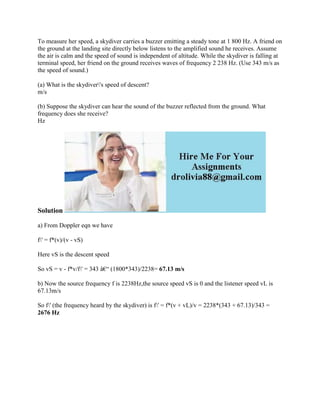Ce diaporama a bien été signalé.
Le téléchargement de votre SlideShare est en cours. ×

# To measure her speed- a skydiver carries a buzzer emitting a steady to.docx

Publicité
Publicité
Publicité
Publicité
Publicité
Publicité
Publicité
Publicité
Publicité
Publicité
PublicitéChargement dans…3
×

## Consultez-les par la suite

1 sur 2 Publicité

# To measure her speed- a skydiver carries a buzzer emitting a steady to.docx

To measure her speed, a skydiver carries a buzzer emitting a steady tone at 1 800 Hz. A friend on the ground at the landing site directly below listens to the amplified sound he receives. Assume the air is calm and the speed of sound is independent of altitude. While the skydiver is falling at terminal speed, her friend on the ground receives waves of frequency 2 238 Hz. (Use 343 m/s as the speed of sound.)
(a) What is the skydiver\'s speed of descent?
m/s

(b) Suppose the skydiver can hear the sound of the buzzer reflected from the ground. What frequency does she receive?
Hz
Solution
a) From Doppler eqn we have
f\' = f*(v)/(v - vS)
Here vS is the descent speed
So vS = v - f*v/f\' = 343 â€“ (1800*343)/2238= 67.13 m/s
b) Now the source frequency f is 2238Hz,the source speed vS is 0 and the listener speed vL is 67.13m/s
So f\' (the frequency heard by the skydiver) is f\' = f*(v + vL)/v = 2238*(343 + 67.13)/343 = 2676 Hz
.

To measure her speed, a skydiver carries a buzzer emitting a steady tone at 1 800 Hz. A friend on the ground at the landing site directly below listens to the amplified sound he receives. Assume the air is calm and the speed of sound is independent of altitude. While the skydiver is falling at terminal speed, her friend on the ground receives waves of frequency 2 238 Hz. (Use 343 m/s as the speed of sound.)
(a) What is the skydiver\'s speed of descent?
m/s

(b) Suppose the skydiver can hear the sound of the buzzer reflected from the ground. What frequency does she receive?
Hz
Solution
a) From Doppler eqn we have
f\' = f*(v)/(v - vS)
Here vS is the descent speed
So vS = v - f*v/f\' = 343 â€“ (1800*343)/2238= 67.13 m/s
b) Now the source frequency f is 2238Hz,the source speed vS is 0 and the listener speed vL is 67.13m/s
So f\' (the frequency heard by the skydiver) is f\' = f*(v + vL)/v = 2238*(343 + 67.13)/343 = 2676 Hz
.

Publicité
Publicité

### To measure her speed- a skydiver carries a buzzer emitting a steady to.docx

1. 1. To measure her speed, a skydiver carries a buzzer emitting a steady tone at 1 800 Hz. A friend on the ground at the landing site directly below listens to the amplified sound he receives. Assume the air is calm and the speed of sound is independent of altitude. While the skydiver is falling at terminal speed, her friend on the ground receives waves of frequency 2 238 Hz. (Use 343 m/s as the speed of sound.) (a) What is the skydiver's speed of descent? m/s (b) Suppose the skydiver can hear the sound of the buzzer reflected from the ground. What frequency does she receive? Hz Solution a) From Doppler eqn we have f' = f*(v)/(v - vS) Here vS is the descent speed So vS = v - f*v/f' = 343 â€“ (1800*343)/2238= 67.13 m/s b) Now the source frequency f is 2238Hz,the source speed vS is 0 and the listener speed vL is 67.13m/s So f' (the frequency heard by the skydiver) is f' = f*(v + vL)/v = 2238*(343 + 67.13)/343 = 2676 Hz